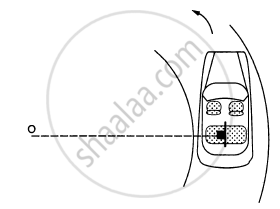Share

# A Car Moving at a Speed of 36 Km/Hr is Taking a Turn on a Circular Road of Radius 50 M. a Small Wooden Plate is Kept on the Seat with Its Plane Perpendicular to the Radius of the Circular Road. - Physics

ConceptCircular Motion

#### Question

A car moving at a speed of 36 km/hr is taking a turn on a circular road of radius 50 m. A small wooden plate is kept on the seat with its plane perpendicular to the radius of the circular road (In the following figure). A small block of mass 100 g is kept on the seat which rests against the plate. the friction coefficient between the block and the plate is. (a) Find the normal contact force exerted by the plate on the block. (b) The plate is slowly turned so that the angle between the normal to the plate and the radius of the road slowly increases. Find the angle at which the block will just start sliding on the plate.#### Solution

Given :
Speed of the car = v = 36 km/h = 10 m/s
Friction coefficient between the block and the plate = μ = 0.58
Mass of the small body = m = 100 g = 0.1 kg

(a) Let us find the normal contact force (N) exerted by the plant of the block.

$N = \frac{\text{mv}^2}{r} = 0 . 1 \times \frac{100}{50} = \frac{1}{5} = 0 . 2$

(b) The plate is turned; so, the angle between the normal to the plate and the radius of the rod slowly increases.
Therefore, we have :

$N = \frac{\text{mv}^2}{r}\text{ cos }\theta . . . \left(\text{ i }\right)$

$\mu N = \frac{m v^2}{r}\text{ sin }\theta . . .$(ii)

$\text { On using i and ii , we get : }$

$\frac{\mu m v^2}{r}\cos\theta = \frac{\text{mv}^2}{r}\sin\theta$

$\Rightarrow \mu = \text{ tan }\theta$

$\Rightarrow \theta = \tan^{- 1} (0 . 58) \approx 30^\circ$

Is there an error in this question or solution?

#### APPEARS IN

Solution A Car Moving at a Speed of 36 Km/Hr is Taking a Turn on a Circular Road of Radius 50 M. a Small Wooden Plate is Kept on the Seat with Its Plane Perpendicular to the Radius of the Circular Road. Concept: Circular Motion.
S